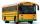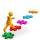# Two cars

From the town A to town B started two cars. The first at 7:00 at average speed 60 km per hour, the second at 10:00 at average speed 100 km per hour. The first car will not stay in B, and on the way back meet the second car at half way from A to B. At what time in hours its will meet?

Result

t = 10:45 h#### Solution:

1.5 s = 60(t-7)
0.5 s = 100(t-10)

1.5s-60t = -420
0.5s-100t = -1000

s = 150
t = 434 = 10.75

Calculated by our linear equations calculator.

Leave us a comment of example and its solution (i.e. if it is still somewhat unclear...):Be the first to comment!#### To solve this verbal math problem are needed these knowledge from mathematics:

Do you have a linear equation or system of equations and looking for its solution? Or do you have quadratic equation? Do you want to convert length units?

## Next similar examples:

1. TruckIn 7 hours started from town Krnov truck at speed 40 km/h. Passenger car started against it in 8 hours 30 minutes from the city of Jihlava at speed 70 km/h. Distance between this two cities is 225 km. At what time and at what distance from Krnov this two.
2. Two citiesCities A and B are 200 km away. At 7 o'clock from city A, the car started at an average speed of 80 km/h, and from B at 45 min later the motorcycle is started at an average speed of 120 km/h. How long will they meet and at what distance from the point A it
3. Cycling tripAt 8 am started a group of children from the camp to day cycling trip. After 9:00 weather deteriorated sharply and the leader decided to send for the children bus on the same route, which started at 10 hours. For how long and at what distance from the camp
4. RavensOn two trees sitting 17 ravens. If 3 ravens flew from first to second tree and 5 ravens took off from second tree then the first tree has 2 times more ravens than second tree. How many ravens was originally on every tree?
5. Journey to schoolOn the way to school I went steady step. In the first half of the journey, I counted every second step, in the second half every third. How many steps I do when I went to school if I counted double step are 250 more than three steps?
6. FamilyFamily has 4 children. Ondra is 3 years older than Matthew and Karlos 5 years older than the youngest Jane. We know that they are together 30 years and 3 years ago they were together 19 years. Determine how old the children are.
7. Fifth of the numberThe fifth of the number is by 24 less than that number. What is the number?
8. Ages 2A man's age is 4 times his son's age. After 5 years he will be just twice his son's age, find their ages.
9. Substitutionsolve equations by substitution: x+y= 11 y=5x-25
10. ATC campThe owner of the campsite offers 79 places in 22 cabins. How many of them are triple and quadruple?
11. Father and sonWhen I was 11, my father was 35 years old. Today, my father has three times more years than me. How old is she?
12. TheatroTheatrical performance was attended by 480 spectators. Women were in the audience 40 more than men and children 60 less than half of adult spectators. How many men, women and children attended a theater performance?
13. MushroomsEva and Jane collected 114 mushrooms together. Eve found twice as much as Jane. How many mushrooms found each of them?
14. Six yearsIn six years Jan will be twice as old as he was six years ago. How old is he?
15. Linear systemSolve a set of two equations of two unknowns: 1.5x+1.2y=0.6 0.8x-0.2y=2
16. Three figures - numbersThe sum of three numbers, if each is 10% larger than the previous one, is 662. Determine the figures.
17. Lee isLee is 8 years more than twice Park's age, 4 years ago, Lee was three times as old. How old was Lee 4 years ago?